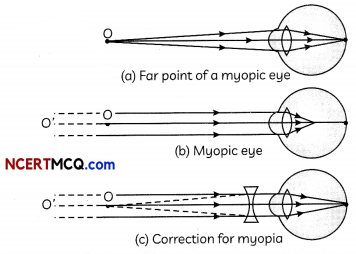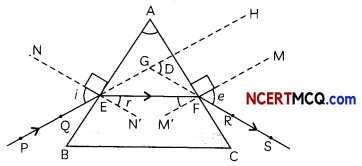## Defects of Vision

Sometimes, the eye may gradually lose its power of accommodation due to which the person cannot see the objects distinctly and comfortably. The defects of vision are described below:

## Myopia

Myopia is also known as near-sightedness. A person can see nearby objects clearly but cannot see distant objects clearly as the image of the object is formed in front of the retina.

Causes of Myopia

1. Excessive curvature of the cornea
2. Elongation of the eyeball

Correction of Myopia
Myopia be corrected by using a concave (diverging) lens of appropriate focal Iength (or Power):Example 1.
The far point of a myopic person is 80 cm in front of the eye. What is the nature and power of the lens required to correct the problem?
To find the nature and power of lens, we will use u = ∞, v = -80 cm.
$$\frac{1}{f}=\frac{1}{v}-\frac{1}{u}=-\frac{1}{80}$$ ⇒ f = – 80cm = – 0.8m
It is a concave lens of focal length 0.8 m. Power of lens = -1.25 D.

## Correction of Hypermetropia

Hypermetropia can be corrected by using a convex (converging) lens of appropriate focal length (or Power).Example 2.
A person needs a lens of power -5.5 dioptres for correcting his distant vision. For correcting his near vision he needs a Lens of power +1.5 dioptre. What is the focal length of the lens required for correcting (A) distant vision, and (B) near vision?
The relation between power (P) and focal
(fin metres) is given by P = $$\frac{1}{f(\text { in } m)}$$ length

(A) To find the focal length of the lens required for correcting distant vision, it is given that P = -5.5 D.
Therefore, using the reLation
P = $$\frac{1}{f(\text { in } m)}$$
⇒ f(in m) = $$\frac{1}{P}=+\frac{1}{5.5}$$
= + 0.67 m = + 66.67 cm
It is a concave lens of focal length 18 cm.

(B) To find the focal length of the lens required for correcting near vision, it is given that P = + 1.5 D.
Therefore, using the relation
P = $$\frac{1}{f(\text { in } m)}$$
⇒ f (in m)
= + 0.67 m = + 66.67 cm
It is a convex Lens of focal Length 66.67 cm.

## Presbyopia

With ageing, the near point of most people gradually recedes due to which they are unable to see nearby objects distinctly. A person may suffer from both myopia and hypermetropia

Causes of Presbyopia

1. Gradual weakening of the ciliary muscles with age
2. Decreasing flexibility of the crystalline lens.

Correction of Presbyopia
For a person suffering from both myopia and hypermetropia, bi-focal lenses are required in which the upper part is a concave lens and lower part is a convex lens.Example 3.
The human eye can focus objects at different distances by adjusting the focal length of the eye lens. This is due to
(a) presbyopia.
(b) accommodation.
(c) near-sightedness.
(d) far-sightedness.
(b) accommodation.

Explanation: The ability of the human eye to focus on nearby and distant objects by changing the curvature and hence focal length of the eye lens is called accommodation of the eye.

## Refraction Of Light Through A Prism

A prism has two triangular bases and three rectangular lateral surfaces which are inclined to each other. The angle between its two lateral faces is called the angle of the prism. In the figure,
PE – Incident ray ∠i – Angle of incidence
EF – Refracted ray ∠r – Angle of refraction
FS – Emergent ray ∠e – Angle of emergence
∠A – Angle of the prism ∠D – Angle of deviation